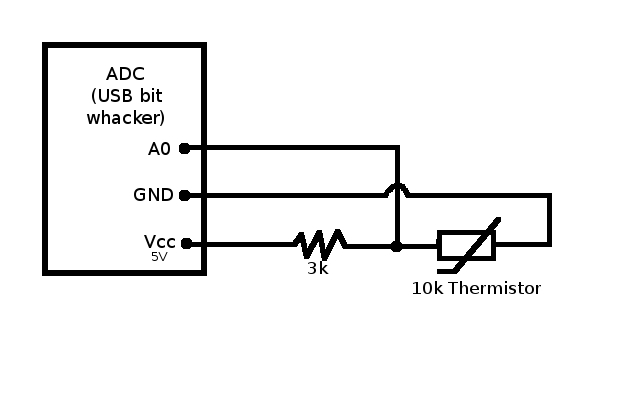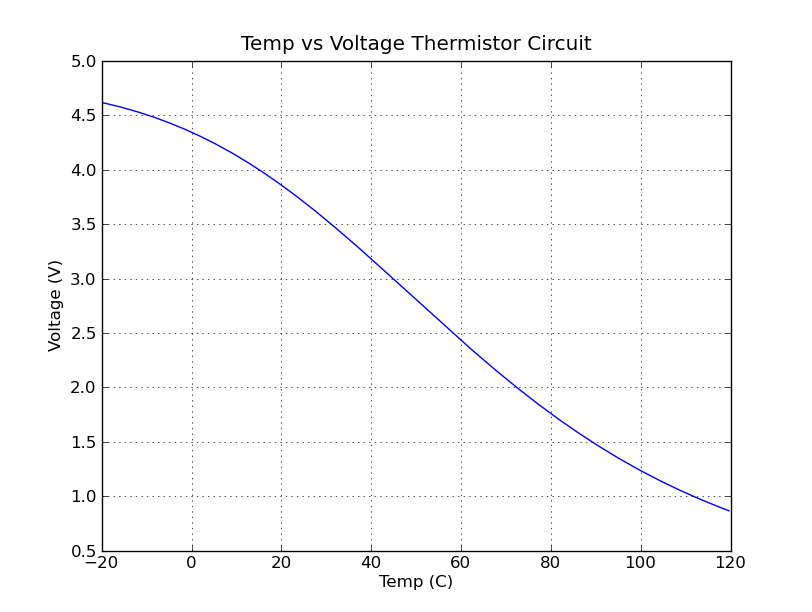An exploration of how to read a read a thermistor (a temperature monitor) by measuring the thermistor’s resistance using an analog input link on a USB Bit Wacker (UBW).

# Overview

I have been struggling over the past week to come up with a solution to read a 10k NTC thermistor for my work. I eventually purchased a USB Bit Whacker (UBW) to perform my A/D conversion as well as satisfy my needs for gpio. Hardware in hand I had to figure out how to measure resistance with a analog input.

A friend of mine recommended that I use an LM317L to regulate a constant current and just measure the voltage through the thermistor. While this is a good idea for small resistances to read a 10k thermistor I may be looking at as much as 15k ohm resistance for low temperatures. Therefore if the current cannot be regulated at a very small very precise amount then there will be a problem of producing voltage exceeding the 5V tolerance of the A/D card (I would need like <.0003 A or something). I considered putting a resistor in parallel with the thermistor to limit the resistance and prevent voltage that will damage my A/D card. But when calculating the temperature from the resistance from the voltage any extra steps introduce measurement errors that begin to multiply and add up. This led to low accuracy resistance/temp readings.

After exhausting this option I came across a very simple solution to this problem using a voltage divider. Since the UBW has a 5V VCC line I just used a simple voltage divider with the thermistor to create a voltage between 0-5V that is proportional to the resistance to the thermistor. Here is the schematics and the Temp Vs. Voltage graph:This solution was significantly better. It was very simple to implement requiring only one resistor (I don’t even need a separate power source or current regulator). I could measure within 10ohms of the resistance that the meter measured. NOTE: This accuracy highly dependent on the accuracy of the measured voltage which sometimes was accurate and sometimes wasn’t do to the variance in the Vref from the USB. At home I measured it at 4.8V, at work 5.0V. If this isn’t set correctly in the software that converts the 12bit analog value into a voltage then the voltage measurement will be off, and so will the resistance/temp measurement.

All in all I am very pleased with the UBW and am looking to add USART support and SPI to the firmware provided on the UBW Project Page. I’ll post my results here.